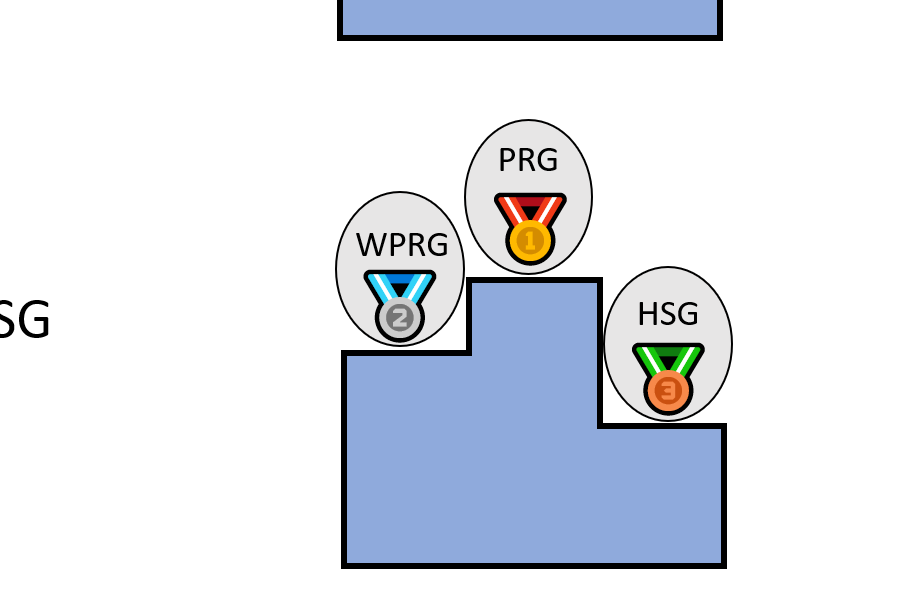Back to list of papers

# Derandomizing Space-Bounded Computation via Pseudorandom Generators and their Generalizations

PhD dissertation by William M. Hoza

Abstract (for specialists)

To what extent is randomness necessary for efficient computation? We study the problem of deterministically simulating randomized algorithms with low space overhead. The traditional approach to this problem is to try to design sufficiently powerful pseudorandom generators (PRGs). PRG constructions often provide deep insights into models of computation and have applications beyond derandomization. There are other approaches based on generalizations of the PRG concept that are potentially easier to construct yet still valuable for derandomization. One such generalization is a hitting set generator (HSG), a standard "one-sided" version of a PRG. Another such generalization, introduced recently by Braverman, Cohen, and Garg (SICOMP 2020), is a weighted pseudorandom generator (WPRG), which is similar to a PRG except probability distributions are replaced by "pseudodistributions." In this dissertation, we present new results (obtained jointly with collaborators) regarding all three of these approaches to derandomizing space-bounded algorithms.

Regarding the PRG approach, we present improved PRGs for interesting models of computation including unbounded-width permutation branching programs, read-once $$\mathbf{AC}^0$$ formulas, and superlinear-size constant-depth threshold circuits. The first two PRGs are unconditionally near-optimal; substantially improving the third would require a breakthrough in circuit lower bounds. Each PRG is in some way connected to derandomizing space-bounded algorithms.

Regarding generalizations of PRGs, we present new constructions and applications of HSGs and WPRGs for read-once branching programs (ROBPs), the nonuniform model that directly corresponds to randomized space-bounded algorithms. In particular, we construct explicit HSGs and WPRGs with an optimal dependence on the error parameter $$\epsilon$$. In terms of applications, we show that optimal HSGs for polynomial-width ROBPs could be used to derandomize decision algorithms with two-sided error ($$\mathbf{BPL}$$, not just $$\mathbf{RL}$$). Unconditionally, we explain how to use recent WPRG constructions to deterministically simulate randomized space-$$S$$ decision algorithms in space $$O\left(S^{3/2} / \sqrt{\log S}\right)$$, a slight improvement over Saks and Zhou's celebrated $$O(S^{3/2})$$ bound (JCSS 1999). Finally, using the techniques underlying our HSG construction, we give an improved unconditional derandomization of log-space algorithms that have one-sided error and low success probability.

Not-so-abstract (for curious outsiders)

⚠️ This summary might gloss over some important details.

A "randomized" algorithm tosses coins to make decisions, whereas a "deterministic" algorithm doesn't use any randomness. In practice, randomized algorithms are often more efficient than deterministic algorithms. However, all else being equal, deterministic algorithms are preferable, because we don't always have access to random bits. "Derandomization" is the art of converting randomized algorithms into deterministic algorithms without making them significantly worse in other respects.

For several decades now, theoretical computer scientists have been trying to prove that every algorithm can be derandomized without significantly increasing the amount of memory that the algorithm uses. That is, given an arbitrary randomized algorithm that uses $$S$$ bits of memory, we would like to convert it into a deterministic algorithm that uses $$S'$$ bits of memory, where $$S'$$ is only slightly larger than $$S$$. I worked on this problem for my PhD and made some progress on it (with collaborators). For example, my dissertation explains a method of derandomization where the value $$S'$$ is slightly smaller than what was known before (but still significantly larger than $$S$$, unfortunately).

My oral defense was in June 2021; the dissertation was published online in October 2021.

Expository material:Slides from my PhD defense (June 2021). See also these slides from my PhD proposal (August 2020).

☢️ Errata:

• (October 2021) The proof of Lemma 5.2.16 is not correct. See Lemma 17 in this paper for a corrected version.